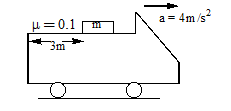Kinetic friction
Question

# A block of mass m rests on truck accelerating at 4 $m/{s}^{2}$. Block of mass m is 3m away from end of the truck. If coefficient of friction between block & truck surface is $\mu =0.1$ , the time taken by block to fall off the truck isModerate
Solution

## $\begin{array}{l}{S}_{rel}={U}_{rel}\text{\hspace{0.17em}}t+\frac{1}{2}{a}_{rel}\text{\hspace{0.17em}}{t}^{2}\\ 3=0t+\frac{1}{2}\left(4-1\right){t}^{2}\\ t=\sqrt{2}s\end{array}$

Get Instant Solutions